# Test: Properties of Isosceles Triangle

## 10 Questions MCQ Test Mathematics (Maths) Class 9 | Test: Properties of Isosceles Triangle

Description
Attempt Test: Properties of Isosceles Triangle | 10 questions in 10 minutes | Mock test for Class 9 preparation | Free important questions MCQ to study Mathematics (Maths) Class 9 for Class 9 Exam | Download free PDF with solutions
QUESTION: 1

### In two right triangles, one side and an acute angle of one are equal to the corresponding side and angle of the other, then ΔABC ≅ ΔDEF by the criterion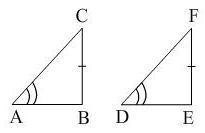Solution: Answer is B because in this it is given that angle A = D . Then also B=E as they are right angle. And finally line CB = line FE (given) there for the criteria is AAS.
QUESTION: 2

### In ΔABC if ∠A = ∠B, then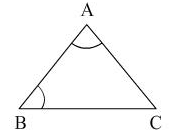Solution: Becuase Sides opposite to equal angles are also equal.angle A = angle BBC is opposite to angle A and AC is opposite to angle B.Therefore, AC=BC in triangle ABC.
QUESTION: 3

### In the given figure, AB = AC and ∠ACD = 105°, ∠BAC will be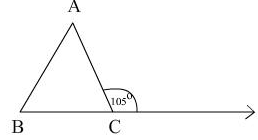Solution:

Angle acb = 75 ( linear pair) Ab= Ac hence angle B= angle c in triangle ABC angle a+B+c= 180. angle B+ c = 150 hence angle c a= 30

QUESTION: 4

PQR is a triangle in which PQ = PR and S is any point on the side PQ. Through S, a line is drawn parallel to QR and intersecting PR at T. then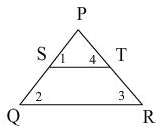Solution:
QUESTION: 5

The altitude of an equilateral triangle of side a to any of its other sides from the opposite vertex is

Solution:
QUESTION: 6

In an isosceles right angled triangle, the measures of the acute angles are​

Solution:
QUESTION: 7

In ΔABC if AB = BC then:

Solution:
QUESTION: 8

Pick out the incorrect statement

Solution:

The altitudes of a triangle are the line segments drawn from each side of the triangle to the angle opposite that side, such that the line segment is perpendicular to the side it is drawn from. In an isosceles triangle, only the altitudes of the legs of equal length in the triangle are congruent.

QUESTION: 9

If the bisector of the exterior vertical angle of a triangle is parallel to the base, then it is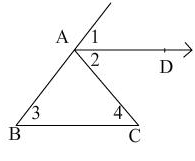Solution:
∠CAE is the external vertical angle and AD is its bisector.

∴ ∠CAD = ∠DAE  .... (i)

∴ ∠CAD = ∠ACB  .... (ii)  (alternate angle)

and ∠DAE = ∠ABC  ....(iii)  (corresponding angle)

From (i), (ii) and (iii),

∠ABC = ∠ACB

∴ AC = AB  (opposite sides to equal angles)

Hence, ΔABC is isosceles.
QUESTION: 10

In PQR, PQ = PR and R = 65°, then P = ?

Solution:

By using the theorem,If two sides of a triangle are equal then the opposite angles to the sides are equal.
⇒ if PQ=PR then ∠Q=∠R
in triangle PQR,
⇒ ∠P+∠Q+∠R=180°
⇒ ∠P+∠Q+∠Q=180° (∵∠Q=∠R)
⇒ ∠P+65°+65°=180°
⇒ ∠P+130°=180°
⇒ ∠P=180°-130°
⇒ ∠P=50Use Code STAYHOME200 and get INR 200 additional OFF Use Coupon Code